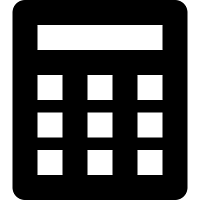calculator program in c using functionsHere we will discuss about how to make calculator using C Program. Wap to write a program in c to make calculator by using function.calculator program in c using functions

#include<stdio.h>
#include<conio.h>
#include<math.h>
int sum(int,int);
int product(int,int);
int subtract(int,int);
void main()
{
clrscr();
int a,b,result=0;
char op;
printf("Enter 1st number, operator and 2nd number ");
scanf("%d %c %d",&a,&op,&b);
if (op=='+')
{
result=sum(a,b);
printf("sum = %d",result);
}
if (op=='*')
{result=product(a,b);
printf("product = %d",result);
}
if (op=='-')
{
result=subtract(a,b);
printf("subtraction = %d ",result);
}
getch();
}
int sum(int a,int b)
{return a+b;
}
int product(int a,int b)
{
return a*b;
}
int subtract(int a,int b)
{
return a-b;
}